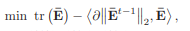# How to express ||E||2

How to express the first order Taylor expansion of matrix E norm with cvxDo you know how to compute this with an explicit formula in “regular”, i.e… double precision MATLAB. That’s the first step. The second step the objective you want to minimize is convex.

is that the operator 2-norm? If so, I think the gradient of the norm involves the singular vector corresponding to the maximum singular value. I doubt there’s anything convex going on there. I will assume if is non-convex unless you show otherwise.